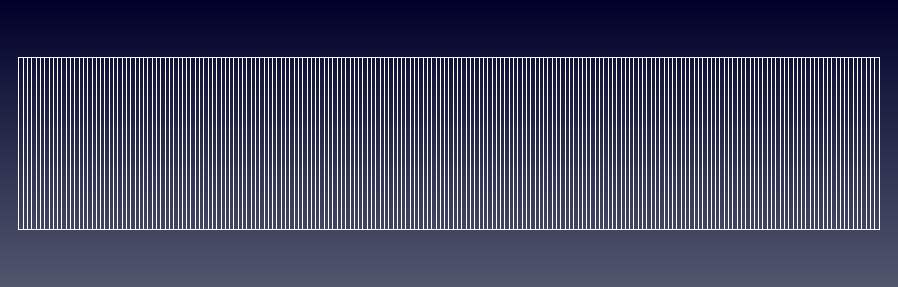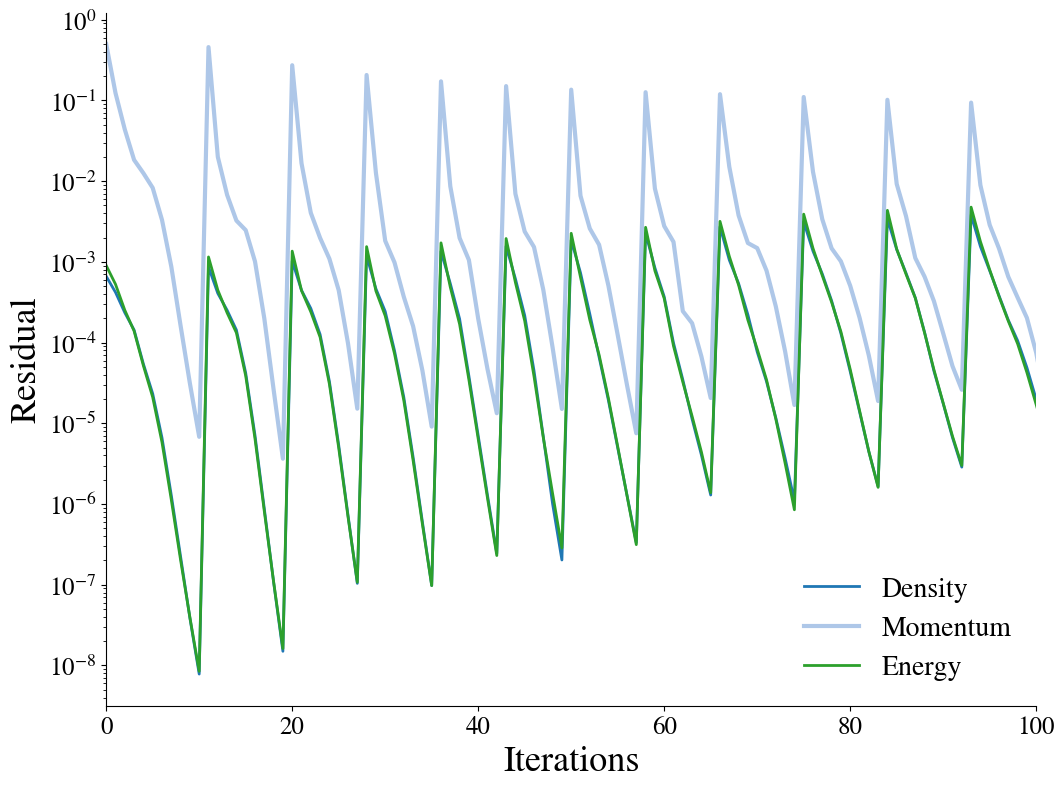In :
import pandas as pd
import numpy as np
import matplotlib as plt
from IPython.display import Image


# Shock Tube¶

This validation case consideres one-dimensional shock propagation. For validation purposes, the study compares the numerical results to an analytical solution.

### Material properties¶

It is assumed the fluid can be treated as an ideal gas for which the following material properties hold:

In :
mP = pd.read_csv('./input/matProp.csv')
mP

Out:
Gas constant Specific heat ratio
0 287.058 1.4

### Initial conditions¶

The following initial conditions are specified:

In :
iCI = pd.read_csv('./input/intialCond.csv')
iCI

Out:
Region Pressure Temperature Velocity
0 0 100000 348.432 0.0
1 1 10000 278.746 0.0

### Grid¶

For the purpose of the analysis a one-dimensional structured mesh is generated using the blockMesh utility. A tube length of 10 m is specified.

In :
from IPython.display import Image, HTML, display
from glob import glob
imagesList=''.join( ["<img style='width: 600px; margin: 10px; float: left; border: 0px solid black;' src='%s' />" % str(s)
for s in sorted(glob('screenShot/mesh/mesh1Far.png')) ])
display(HTML(imagesList))### Running¶

A script is provided to set up the case and run the simulation. The script ./runSim generates the mesh, initialises the two regions and runs the simulation.

### Convergence¶

Below, the convergence of the density, momentum and energy equations' residuals are shown as a function of the number of solver iterations:

In :
from IPython.display import Image, HTML, display
from glob import glob
imagesList=''.join( ["<img style='width: 415px; margin: 10px; float: left; border: 0px solid black;' src='%s' />" % str(s)
for s in sorted(glob('screenShot/results/conv*.png')) ])
display(HTML(imagesList))### Results¶

To validate the numerical results, they are plotted against the analytical solution. The pressure and velocity after 0.007 s are shown respectively:

In :
from IPython.display import Image, HTML, display
from glob import glob
imagesList=''.join( ["<img style='width: 415px; margin: 10px; float: left; border: 0px solid black;' src='%s' />" % str(s)
for s in sorted(glob('screenShot/results/results*.png')) ])
display(HTML(imagesList))In :
from IPython.core.display import HTML

def css_styling():